How Cheenta works to ensure student success?
Explore the Back-Story

# Test of Mathematics Solution Subjective 74 - Sum of Squares of Digits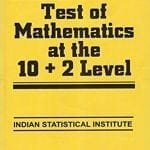This is a Test of Mathematics Solution Subjective 74 (from ISI Entrance). The book, Test of Mathematics at 10+2 Level is Published by East West Press. This problem book is indispensable for the preparation of I.S.I. B.Stat and B.Math Entrance.

Also visit: I.S.I. & C.M.I. Entrance Course of Cheenta

## Problem

The sum of squares of the digits of a three-digit  positive number is 146, while the sum of the two digits in the unit's and the ten's place is 4 times the digit in the hundred's place. Further, when the number is written in the reverse order, it is increased by 297. Find the number.

## Solution

Letis unit's digit,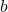is ten's digit and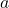is the hundred's digit.

Then from the given information we get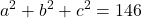... (i)=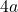...(ii)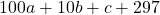=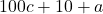...(iii)=From (iii) we get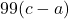=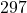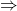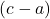=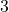Now from (ii) we get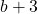=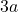=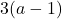So b is a multiple of 3, b can be 0, 3, 6, 9.
For b = 0
not possible as
b = 0a = 1c = 4
then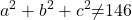Similarly for b = 3a = 2c = 5
& b = 6a = 3c = 6 are not possible.
For b = 9a = 4c = 7So the only solution is 497.This is a Test of Mathematics Solution Subjective 74 (from ISI Entrance). The book, Test of Mathematics at 10+2 Level is Published by East West Press. This problem book is indispensable for the preparation of I.S.I. B.Stat and B.Math Entrance.

Also visit: I.S.I. & C.M.I. Entrance Course of Cheenta

## Problem

The sum of squares of the digits of a three-digit  positive number is 146, while the sum of the two digits in the unit's and the ten's place is 4 times the digit in the hundred's place. Further, when the number is written in the reverse order, it is increased by 297. Find the number.

## Solution

Letis unit's digit,is ten's digit andis the hundred's digit.

Then from the given information we get... (i)=...(ii)=...(iii)=From (iii) we get==Now from (ii) we get==So b is a multiple of 3, b can be 0, 3, 6, 9.
For b = 0
not possible as
b = 0a = 1c = 4
thenSimilarly for b = 3a = 2c = 5
& b = 6a = 3c = 6 are not possible.
For b = 9a = 4c = 7So the only solution is 497.

### Knowledge Partner